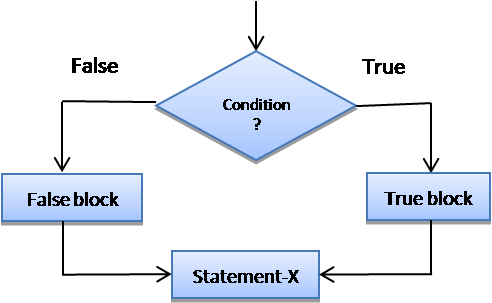• If …else statement is an extension of simple if statement.
• When we have two different path to follow,then simple if statement cannot be useful because if provides a single path to follow.
• In such situation if…else statement is more suitable.
• If…else statement helps to perform different actions based on true or false condition.
• It has the following form:

Syntax:` `

``````if (condition)
{
//true block;
}
else
{
//false block;
}``````• If given expression evaluates to true then true block will be executed and if condition is false then else block will be execute.
• In any situation only one block will be executed that is if condition is true then true block will be executed and else block will be skipped.
• Similarly, if condition is false then else block will be executed and true block will be skipped.
• When if and else statement has a single statement then brackets are not required but it is necessary when it contain two or more statements.
• The above code fragment (part) will display odd or even number depending on the condition.

Example:` `

```// Program to check if number is even or odd.
#include "stdio.h"
#include "conio.h"

void main()
{
int n;
clrscr();

printf("Enter No: ");
scanf("%d",&n);

if(n % 2 == 0)
{
printf("'\n %d Number is even", n);
}
else
{
printf("\n %d Number is odd", n);
}
getch();

}```

### Conditional Statement:

• Conditional statement is written using conditional operator also known as ternary operator.
• The format of the conditional operator is as follow:
``(Condition)? Statement-1 : Statement-2``
• It takes three operands.
• If condition is true then statement-1 will be executed otherwise statement-2 will be executed.
• We can also represent the condition for assignment operation like:
``variable = (Condition)? Value-1 : Value-2``
• Here if condition is true then variable has assigned the value-1 otherwise it has assigned the value-2
``commission = (sales > 50000) ? 2000 : 0;``
• In above example if sale is > 50000 then commission value will be 2000 otherwise it will be zero.
• It is similar to if…else statement and we can rewrite conditional statement using if…else statement as follow:
``````if( sales > 50000)
commission = 2000 ;
else
commission = 0 ;``````